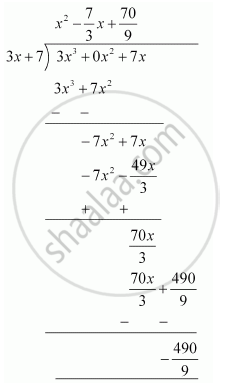# Check whether 7 + 3x is a factor of 3x3 + 7x. - Mathematics

Check whether 7 + 3x is a factor of 3x3 + 7x.

#### Solution 1

7 + 3x will be a factor of 3x3 + 7x only if 7 + 3x divides 3x3 + 7x leaving no remainder.

Let p(x) = 3x3 + 7x

7 + 3x = 0

⇒ 3x = -7

⇒ x = -7/3

therefore"Remainder "= 3(-7/3)^3+7(-7/3)

= -343/9-49/3

= -490/9

≠ 0

∴ 7 + 3x is not a factor of 3x3 + 7x.

#### Solution 2

Let us divide (3x3 + 7x) by (7 + 3x). If the remainder obtained is 0, then 7 + 3will be a factor of 3x3 + 7x.

By long division,As the remainder is not zero, therefore, 7 + 3x is not a factor of 3x3 + 7x.

Concept: Remainder Theorem
Is there an error in this question or solution?
Chapter 2: Polynomials - Exercise 2.3 [Page 40]

#### APPEARS IN

NCERT Class 9 Maths
Chapter 2 Polynomials
Exercise 2.3 | Q 3 | Page 40
Share